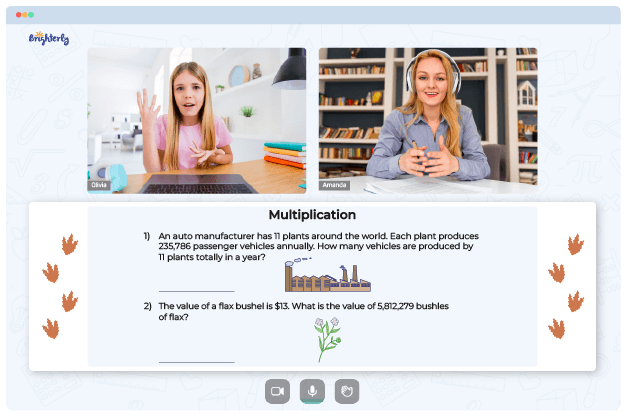# Multiplying and Dividing Rational Expressions Worksheet

The multiplying and dividing rational expressions worksheet is an educational resource that contains a series of math problems focused on multiplying and dividing rational expressions. Our multiply and divide rational expressions worksheet will help students reinforce their knowledge of the concept of multiplying and dividing rational expressions.

## Multiplying and Dividing Rational Expressions

Rational expressions are algebraic expressions that contain fractions with variables in the numerator, denominator, or both. Multiplying and dividing rational expressions involve multiplying or dividing the numerators and denominators of these expressions, similar to how one would multiply or divide fractions.

Math for Kids

Is Your Child Struggling With Math?
1:1 Online Math TutoringThe multiplying and dividing rational expressions worksheet answer key will include problems that require students to simplify expressions, factor polynomials, and find common denominators before performing multiplication or division. The problems will also involve simplifying the resulting expressions by factoring and canceling out common factors.

The algebra 2 multiplying and dividing rational expressions worksheet is appropriate for kids in junior or sophomore year high school math classes. The multiplying and dividing rational expressions worksheet answers comes with practice questions and solutions for students. You can easily download Brighterly’s multiplying and dividing rational expressions worksheet with answers pdf.### Multiplying and Dividing Rational Expressions Worksheet PDF

Multiplying And Dividing Rational Expressions Worksheet### Multiplying and Dividing Rational Expressions Worksheet PDF

Multiplying And Dividing Rational Expressions Worksheet With Answers PDF### Multiplying and Dividing Rational Expressions Worksheet PDF

Multiplying And Dividing Rational Expressions Worksheet Answers### Multiplying and Dividing Rational Expressions Worksheet PDF

Multiplying And Dividing Rational Expressions Worksheet Answer Key

Struggling with Multiplication?• Does your child struggle to understand multiplication concepts?
• Try lessons with an online tutor.

Is your child having trouble mastering multiplication? An online tutor could provide the necessary support.